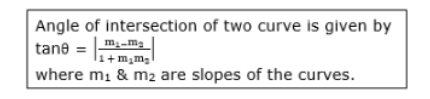Deepak Scored 45->99%ile with Bounce Back Crack Course. You can do it too!

# Find the angle to intersection of the following curves :

Question:

Find the angle to intersection of the following curves :

$2 y^{2}=x^{3}$ and $y^{2}=32 x$

Solution:

Given:

Curves $2 y^{2}=x^{3} \ldots(1)$

$\& y^{2}=32 x$   .....(2)

First curve is $2 y^{2}=x^{3}$

Differentiating above w.r.t $x$,

$\Rightarrow 4 y \cdot \frac{d y}{d x}=3 x^{2}$

$\Rightarrow m_{1}=\frac{d y}{d x}=\frac{3 x^{2}}{4 y} \ldots(3)$

Second curve is $y^{2}=32 x$

$\Rightarrow 2 y \cdot \frac{d y}{d x}=32$

$\Rightarrow y \cdot \frac{d y}{d x}=16$

$\Rightarrow m_{2}=\frac{d y}{d x}=\frac{16}{y} \ldots(4)$

Substituting (2) in (1), we get

$\Rightarrow 2 y^{2}=x^{3}$

$\Rightarrow 2(32 x)=x^{3}$

$\Rightarrow 64 x=x^{3}$

$\Rightarrow x^{3}-64 x=0$

$\Rightarrow x\left(x^{2}-64\right)=0$

$\Rightarrow x=0 \&\left(x^{2}-64\right)=0$

$\Rightarrow x=0 \& \pm 8$

Substituting $x=0 \& x=\pm 8$ in (1) in (2),

$y^{2}=32 x$

when $x=0, y=0$

when $x=8$

$\Rightarrow y^{2}=32 \times 8$

$\Rightarrow y^{2}=256$

$\Rightarrow y=\pm 16$

Substituting above values for $m_{1} \& m_{2}$, we get,

when $x=0, y=16$

$m_{1}=\frac{d y}{d x}$

$\Rightarrow \frac{3 \times 0^{2}}{4 \times 8}=0$

when $x=8, y=16$

$m_{1}=\frac{d y}{d x}$

$\Rightarrow \frac{3 \times 8^{2}}{4 \times 16}=3$

Values of $m_{1}$ is $0 \& 3$

when $x=0, y=0$,

$m_{2}=\frac{d y}{d x}$

$\Rightarrow \frac{16}{y}=\frac{16}{0}=\infty$

when $y=16$

$m_{2}=\frac{d y}{d x}$

$\Rightarrow \frac{16}{y}=\frac{16}{16}=1$

Values of $m_{2}$ is $\infty \& 1$

when $m_{1}=0 \& m_{2}=\infty$

$\Rightarrow \tan \theta=\left|\frac{\mathrm{m}_{1-\mathrm{m}_{2}}}{1+\mathrm{m}_{1} \mathrm{~m}_{2}}\right|$

$\Rightarrow \tan \theta=\left|\frac{\infty-0}{1+\infty \times 0}\right|$

$\Rightarrow \tan \theta=\infty$

$\Rightarrow \theta=\tan ^{-1}(\infty)$

$\therefore \tan ^{-1}(\infty)=\frac{\pi}{2}$

$\Rightarrow \theta=\frac{\pi}{2}$

when $m_{1}=\frac{1}{2} \& m_{2}=2$$\Rightarrow \tan \theta=\left|\frac{3-1}{1+3 \times 1}\right|$

$\Rightarrow \tan \theta=\left|\frac{2}{4}\right|$

$\Rightarrow \tan \theta=\left|\frac{1}{2}\right|$

$\Rightarrow \theta=\tan ^{-1}\left(\frac{1}{2}\right)$

$\Rightarrow \theta \cong 25.516$